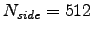Next: polmap Up: GLESP Description and Examples Previous: mappat

# ntot

Description

ntot calculates the number of pixels on a sphere and the size of pixels for a given resolution for both GLESP and HEALPix scheme.

Examples

• ntot -nx 201 -np 402
calculates the total pixel number and size for GLESP scheme at resolution (nx,np)=(201,402) and the corresponding parameters of the nearest allowed resolution for HEALPix scheme.

• ntot -ns 512
calculates the total pixel number and size for HEALPix scheme atand the corresponding parameters of the nearest allowed resolution for GLESP scheme.

• ntot -p 25
calculates the resolution parameters for GLESP scheme for pixel size 25 square arcmin and the corresponding parameters of the nearest allowed resolution and total pixel number for HEALPix scheme.

• ntot -ns 512 -gr N
calculates the total pixel number and the pixel size for HEALPix scheme at N_side=512 and displays the corresponding parameters of the closest allowed resolution in GLESP scheme. Each ring has the same number of pixels.

Verkhodanov Oleg 2009-04-01